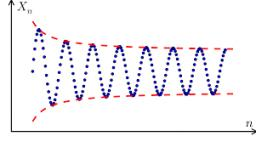# Calculate 5539

Calculate the quotient of the geometric sequence if the sum of the first two terms equals 1.1, and a6 = 10000. A quotient is a natural number.

q =  10

### Step-by-step explanation:Did you find an error or inaccuracy? Feel free to write us. Thank you!